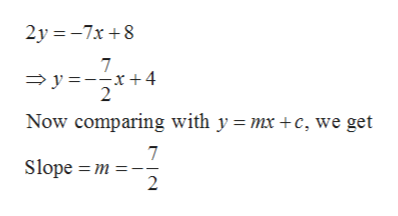# Solve the equation 7x+2y=8 for y, identify the slope, then find an equation for the line that is parallel to the line 7x+2y=8 and passes through the point (0,4).

Question
85 views

Solve the equation 7x+2y=8 for y, identify the slope, then find an equation for the line that is parallel to the line 7x+2y=8 and passes through the point (0,4).

check_circle

Step 1

Given,

Step 2

On simplificatio...help_outlineImage Transcriptionclose2y -7x8 7 y x+4 Now comparing with y mx +c, we get 7 Slope m 2 fullscreen

### Want to see the full answer?

See Solution

#### Want to see this answer and more?

Solutions are written by subject experts who are available 24/7. Questions are typically answered within 1 hour.*

See Solution
*Response times may vary by subject and question.
Tagged in
MathAlgebra

### Other